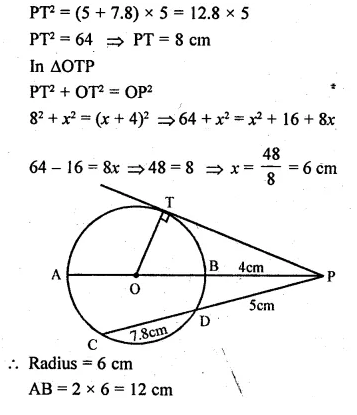Guru

# Question 30(b) In the figure given below, diameter AB and chord CD of a circle meet at P. PT is a tangent to the circle at T. CD = 7.8 cm, PD = 5 cm, PB = 4 cm. Find (i) AB. (ii)the length of tangent PT.

• 1

This is circle based question from Chapter name- circles
Topic – Angle properties of circles
Chapter number- 15

In this question we have that a diameter AB and chord CD of a circle meet at P.

PT is a tangent to the circle at T.

Also some measures are given, CD = 7.8 cm, PD = 5 cm, PB = 4 cm.

Now we have to find (i) AB. (ii)the length of tangent PT.
ICSE Avichal publication
Understanding ICSE Mathematics
Question no 30(b)

Share